Successfully reported this slideshow.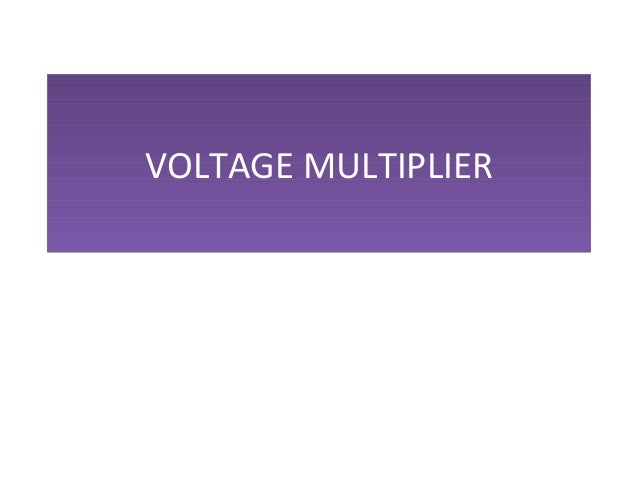Upcoming SlideShare
×

of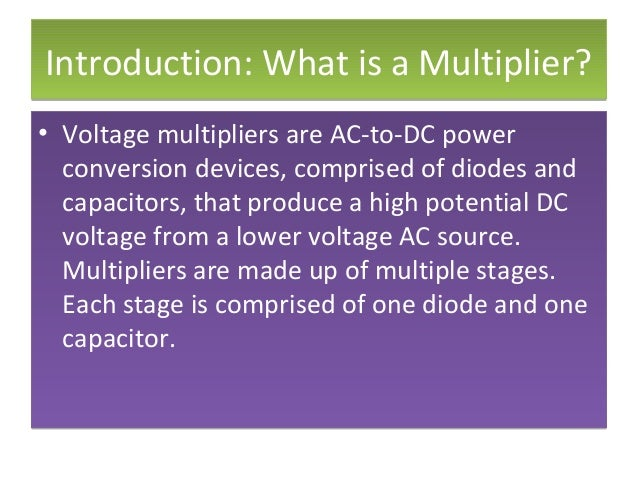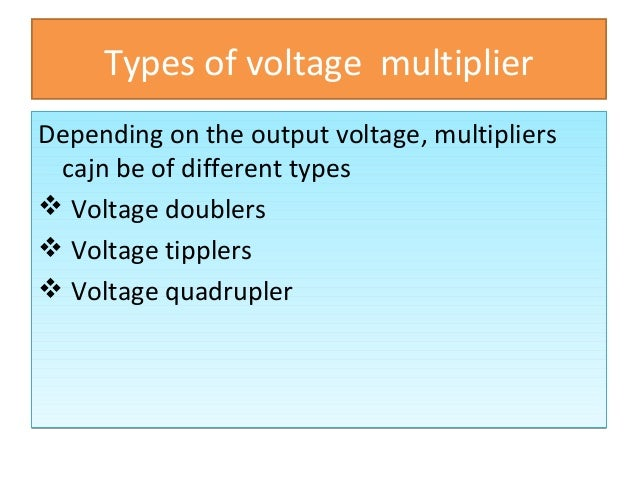Upcoming SlideShare
Voltagedoubler
Next

23

Share

# Voltage multiplier

Voltage multipliers are AC-to-DC power conversion devices, comprised of diodes and capacitors, that produce a high potential DC voltage from a lower voltage AC source. Multipliers are made up of multiple stages. Each stage is comprised of one diode and one capacitor.

See all

See all

### Voltage multiplier

1. 1. VOLTAGE MULTIPLIERVOLTAGE MULTIPLIER
2. 2. Introduction: What is a Multiplier?Introduction: What is a Multiplier? • Voltage multipliers are AC-to-DC power conversion devices, comprised of diodes and capacitors, that produce a high potential DC voltage from a lower voltage AC source. Multipliers are made up of multiple stages. Each stage is comprised of one diode and one capacitor. • Voltage multipliers are AC-to-DC power conversion devices, comprised of diodes and capacitors, that produce a high potential DC voltage from a lower voltage AC source. Multipliers are made up of multiple stages. Each stage is comprised of one diode and one capacitor.
3. 3. Types of voltage multiplier Depending on the output voltage, multipliers cajn be of different types  Voltage doublers  Voltage tipplers  Voltage quadrupler Depending on the output voltage, multipliers cajn be of different types  Voltage doublers  Voltage tipplers  Voltage quadrupler
4. 4. Voltage doublers A Voltage doubler produces a d.c. voltage almost twice the rms value of the input a.c. voltage. Voltage doubler can be of two types; Half wave voltage doubler  Full wave voltage doubler A Voltage doubler produces a d.c. voltage almost twice the rms value of the input a.c. voltage. Voltage doubler can be of two types; Half wave voltage doubler  Full wave voltage doubler
5. 5. Half wave voltage doublerHalf wave voltage doubler The circuit for a half wave voltage doubler. During the positive half cycle of the secondary voltage diode D1 conducts and D2 is cut off. Now capacitor C1 charges to the peak rectified voltage Vm, with polarity shown in the figure. During the negative half cycle, the secondary voltage comes in series with voltage across the capacitor C1. The circuit for a half wave voltage doubler. During the positive half cycle of the secondary voltage diode D1 conducts and D2 is cut off. Now capacitor C1 charges to the peak rectified voltage Vm, with polarity shown in the figure. During the negative half cycle, the secondary voltage comes in series with voltage across the capacitor C1.
6. 6. Contd…Contd… Thus C2 will try to charge towards 2Vm(Vm of the input and Vm of the capacitor C1. After few cycles the voltage across the capacitor C2 will be equal to 2Vm Since diode D2 acts as a short during the negative half-cycle (and diode D1 is open), we can sum the voltages around the outside loop. i.e; −𝑉𝑉 − 𝑉𝑉1−𝑉𝑉2=0 (or) −𝑉𝑉 − 𝑉𝑉 −𝑉𝑉2=0 from which, 𝑉𝑉2=2𝑉𝑉 Thus C2 will try to charge towards 2Vm(Vm of the input and Vm of the capacitor C1. After few cycles the voltage across the capacitor C2 will be equal to 2Vm Since diode D2 acts as a short during the negative half-cycle (and diode D1 is open), we can sum the voltages around the outside loop. i.e; −𝑉𝑉 − 𝑉𝑉1−𝑉𝑉2=0 (or) −𝑉𝑉 − 𝑉𝑉 −𝑉𝑉2=0 from which, 𝑉𝑉2=2𝑉𝑉
7. 7. Circuit Diagram
8. 8. Contd…. In the circuit capacitor C1 will discharge in the negative half cycle. Again in the positive half cycle, it starts charging. Thus the half wave voltage doubler supplies the voltage to the load in one half cycles. Therefore regulation of the half wave voltage doubler is poor. In the circuit capacitor C1 will discharge in the negative half cycle. Again in the positive half cycle, it starts charging. Thus the half wave voltage doubler supplies the voltage to the load in one half cycles. Therefore regulation of the half wave voltage doubler is poor.
9. 9. Full wave voltage doublerFull wave voltage doubler Another voltage doubler circuit called full wave voltage doubler is shown in fig. During the positive half cycle of the secondary voltage diode D1conducts, charging the capacitor the capacitor C1to the peak voltage Vm. At this time diode D2 is non-conducting. During negative half cycle diode D2conducts, charging capacitor C2to Vm, with polarity as marked, while diode D2is non- conducting. Since both capacitors C1and C2are in series, the final output voltage is approximately 2Vm. This circuit is called full wave voltage doubler because one of the output capacitor is being charged during each half cycle of the input voltage Another voltage doubler circuit called full wave voltage doubler is shown in fig. During the positive half cycle of the secondary voltage diode D1conducts, charging the capacitor the capacitor C1to the peak voltage Vm. At this time diode D2 is non-conducting. During negative half cycle diode D2conducts, charging capacitor C2to Vm, with polarity as marked, while diode D2is non- conducting. Since both capacitors C1and C2are in series, the final output voltage is approximately 2Vm. This circuit is called full wave voltage doubler because one of the output capacitor is being charged during each half cycle of the input voltage
10. 10. Circuit diagram
11. 11. Voltage tripler • The voltage tripler arrangement adds another diode/capacitor set. • + half-cycle: C1 charges to Vp through D1, • - half-cycle: C2 charges to 2Vp through C2, • Next + half-cycle: C3 charges to 2Vp through C3. • Output is across C1 & C3. • The voltage tripler arrangement adds another diode/capacitor set. • + half-cycle: C1 charges to Vp through D1, • - half-cycle: C2 charges to 2Vp through C2, • Next + half-cycle: C3 charges to 2Vp through C3. • Output is across C1 & C3.
12. 12. Circuit diagram
13. 13. Voltage QuadruplersVoltage Quadruplers • The voltage tripler arrangement adds another diode/capacitor set. • + half-cycle: C1 charges to Vp through D1, • - half-cycle: C2 charges to 2Vp through C2, • Next + half-cycle: C3 charges to 2Vp through C3. • Next - half-cycle: C4 charges to 2Vp through C4 • Quadruple Output is across C2 & C4. • The voltage tripler arrangement adds another diode/capacitor set. • + half-cycle: C1 charges to Vp through D1, • - half-cycle: C2 charges to 2Vp through C2, • Next + half-cycle: C3 charges to 2Vp through C3. • Next - half-cycle: C4 charges to 2Vp through C4 • Quadruple Output is across C2 & C4.
14. 14. Circuit diagram
15. 15. Common Multiplier Applications Originally used for television CRT's, voltage multipliers are now used for lasers, x-ray systems, traveling wave tubes (TWT's), photomultiplier tubes, ion pumps, electrostatic systems, copy machines, and many other applications that utilizehigh voltage DC. Originally used for television CRT's, voltage multipliers are now used for lasers, x-ray systems, traveling wave tubes (TWT's), photomultiplier tubes, ion pumps, electrostatic systems, copy machines, and many other applications that utilizehigh voltage DC.
•#### Cclkelal

Sep. 23, 2021
•#### ssuserb314bd1

Jul. 29, 2021
•#### BiancaPaulineReyes

Jun. 9, 2020
•#### KaranDhapa1

May. 28, 2020
•#### KyawHein27

May. 23, 2020
•Nov. 1, 2019
•#### AiaNyllCapricho

Sep. 23, 2019
•Aug. 29, 2019
•#### drishtisharan

Aug. 23, 2019
•#### SachinRawat62

Dec. 3, 2018
•#### 1501009029

Feb. 26, 2018
•Feb. 7, 2018
•#### DommyzzeyzooJozeph

Nov. 11, 2017
•#### KanakKaletha

Oct. 29, 2017
•#### AsifaKhanKhann

Jun. 15, 2017
•#### VIAY2

May. 16, 2017
•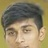#### yashu01

Apr. 6, 2017
•#### MonikaMickey

Jan. 29, 2017
•#### 97151678886

Jan. 28, 2017
•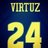#### rimar27

Jan. 19, 2017

Total views

9,601

On Slideshare

0

From embeds

0

Number of embeds

29

638

Shares

0